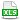HomeTechniques and Tips@RISK DistributionsSensitivity Simulation with RiskSimtable for Specific Values

# 3.40. Sensitivity Simulation with RiskSimtable for Specific Values

Applies to: @RISK 5.x–7.x

As you know, @RISK sensitivity analysis lets you see the impact of uncertain model parameters on your results. But what if some of the uncertain model parameters are under your control? In this case the value a variable will take is not random, but can be set by you. For example, you might need to choose between some possible prices you could charge, different possible raw materials you could use or from a set of possible bids or bets. To properly analyze your model, you need to run a simulation at each possible value for the "user-controlled" variables and compare the results. A Sensitivity Simulation in @RISK allows you to quickly and easily do this, offering a powerful analysis technique for selecting between available alternatives.

In @RISK, any number of simulations can be included in a single Sensitivity Simulation. The RiskSimtable( ) function is used to enter lists of values, which will be used in the individual simulations, into your worksheet cells and formulas. @RISK will automatically process and display the results from each of the individual simulations together, allowing easy comparison.

To run a Sensitivity Simulation:

1. Enter the lists of values you want used in each of the individual simulations into your cells and formulas using RiskSimtable( ). For example, possible price levels might be entered into Cell B2, like this:

=RiskSimtable({100,200,300,400})

This will cause simulation #1 to use a value of 100 for price, simulation #2 to use a value of 200, simulation #3 to use a value of 300 and simulation #4 to use a value of 400. (If you have too many values to place comfortably in the formula, see Cell References in Distributions.)

2. Set the number of simulations in the Simulation Settings dialog box (in this example, 4 simulations) and run the Sensitivity Simulation using the Start Simulation command.

Each simulation executes the same number of iterations and collects data from the same specified output ranges. Each simulation, however, uses a different value from the RiskSimtable( ) functions in your worksheet.

@RISK processes Sensitivity Simulation data just as it processes data from a single simulation. Each output cell for which data was collected has a distribution for each simulation. Using the functions of @RISK, you can compare the results of the different alternatives or scenarios described by each individual simulation. The Distribution Summary graph summarizes how the results for an output range change. There is a different summary graph for each output range in each simulation, and these graphs can be compared to show the differences between individual simulations. In addition, the Simulation Summary report is useful for comparing results across multiple simulations.

The values entered in the RiskSimtable function can be distribution functions, so you can also use Sensitivity Simulation to see how different distribution functions affect your results. For example, you may wish to see how your results change if you alternately try RiskTriang( ), RiskPert( ), or RiskNormal( ) as the distribution type in a given cell. For more, see RiskSimtable with Distributions as Arguments.

Caution:
It is important to distinguish between controlled changes by simulation (which are modeled with the RiskSimtable( ) function), and random variation within a single simulation (which is modeled with distribution functions). RiskSimtable( ) should not be substituted for RiskDiscrete( ) when evaluating different possible random discrete events. Most modeling situations are a combination of random, uncertain variables and uncertain but "controllable" variables. Typically, the controllable variables will eventually be set to a specific value by the user, based on the comparison conducted with a Sensitivity Simulation.

Caution:
Each simulation executed when the number of simulations is greater than one in the Simulation Settings uses the same random number generator seed value. This isolates the differences between simulations to only the changes in the values returned by RiskSimtable( ) functions. If you wish to override this setting, select Multiple Simulations Use Different Seed Values in the Random Number Generator section of the Sampling tab prior to running multiple simulations.

•SenSim.xls